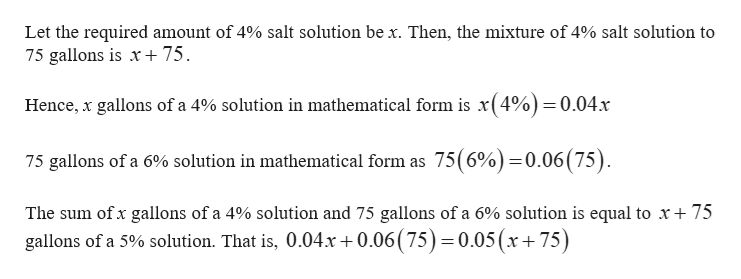# How many gallons of a 4% salt solution must be mixed with 75 gallons of a 6% solution to obtain a 5% solution?

Question
3 views

How many gallons of a 4% salt solution must be mixed with 75 gallons of a 6% solution to obtain a 5% solution?

check_circle

Step 1help_outlineImage TranscriptioncloseLet the required amount of 4% salt solution be x. Then, the mixture of 4% salt solution to 75 75 gallons is x Hence, x gallons of a 4% solution in mathematical form is x(4%)=0.04x 75 gallons of a 6% solution in mathematical form as 75(6%)=0.06(75). The sum ofx gallons of a 4% solution and 75 gallons of a 6% solution is equal to x+ 75 gallons of a 5% solution. That is, 0.04x+0.06 ( 75) 0.05(x+75) fullscreen

### Want to see the full answer?

See Solution

#### Want to see this answer and more?

Solutions are written by subject experts who are available 24/7. Questions are typically answered within 1 hour.*

See Solution
*Response times may vary by subject and question.
Tagged in

### Other